• 个dataframe里面对于两个做分组，最后算个value对应于这两个的分组来算值的矩阵，这个矩阵的行为其中其中。 新建个df import pandas as pd import numpy as np v = [1, 2, 3, 3, 3] a...
前言
主要想做的事情用大白话来讲就是： 一个dataframe里面对于两个列做分组，最后算一个value对应于这两个列的分组来算值的矩阵，这个矩阵的行为其中一个列，列也为其中一个列。
新建一个df
import pandas as pd
import numpy as np
v = [1, 2, 3, 3, 3]
a = pd.DataFrame({'v': v})
d = [2 , 4, 4, 5, 4]
a['d'] = d
c = ['c' , 'h', 'd', 'e',  'c']
a['c'] = c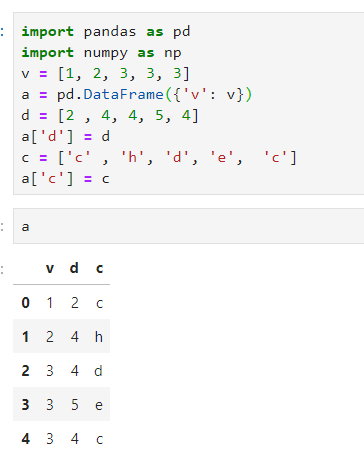df用两列进行分组grouby
a.groupby(['v', 'd'])['c'].count()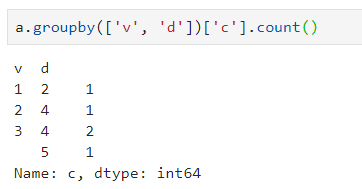我的要干的事情 就是把这个v当作列index，d当作行columns，之后把对应的分组的’c‘.count()放到对应的索引loc处，不存在的值用0填补。
最后得到一个以v为index， d为column的df，也可以搞成对应的矩阵matrix
第一种方法：pd.crosstab
cpd = pd.crosstab(a['v'], a['d'], a['c'], aggfunc='count')
cpd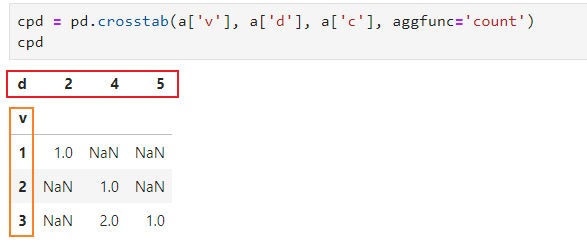查看index和columns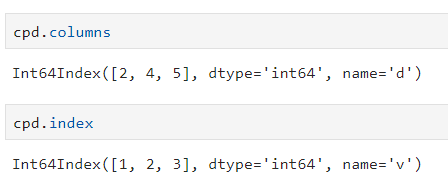我们再来一步就可以得到我们想要的了，把nan填充一下。
cpd = cpd.fillna(0)
cpd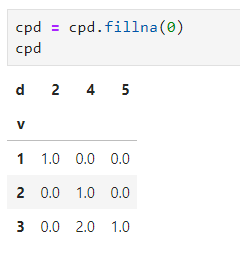转换为array和list的方法
cpd_arr1 = np.array(cpd)
cpd_arr2 = np.array(cpd.values.tolist())
cpd_arr3 = cpd.as_matrix()
cpd_list = cpd.values.tolist()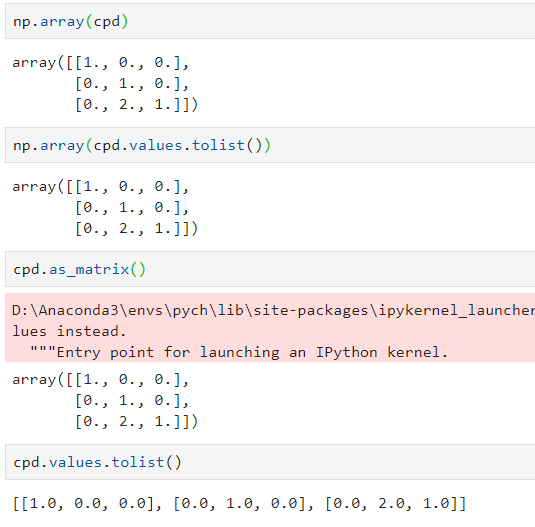整体流程
cpd = pd.crosstab(a['v'], a['d'], a['c'], aggfunc='count')
cpd = cpd.fillna(0)
cpd_arr = np.array(cpd)
cpd_arr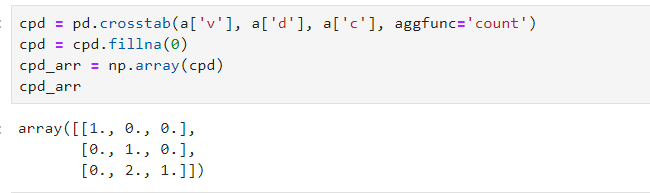总结一句话
np.array(pd.crosstab(a['v'], a['d'], a['c'], aggfunc='count').fillna(0))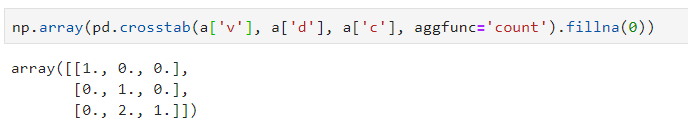第二种方法：pivot()
groupby的as_index=False
a.groupby(['v', 'd'], as_index=False)['c'].count()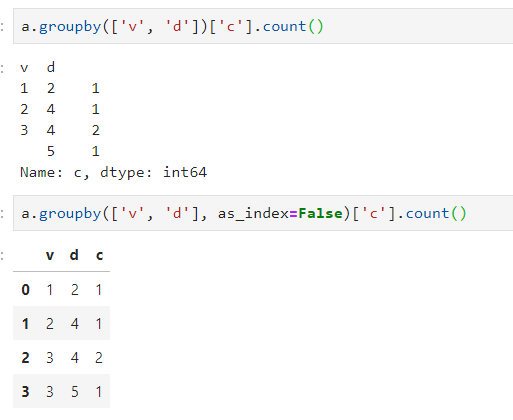把操作的三列的值恢复到df上，每一个列还是对应的列。
pivot
a.groupby(['v', 'd'], as_index=False)['c'].count().pivot('v', 'd', 'c')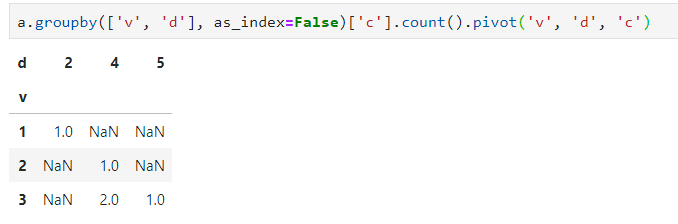对应关系如下：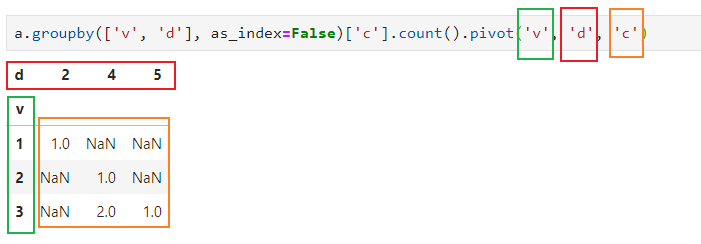整体流程
ppd = a.groupby(['v', 'd'], as_index=False)['c'].count().pivot('v', 'd', 'c')
ppd = ppd.fillna(0)
ppd_arr = np.array(ppd)
ppd_arr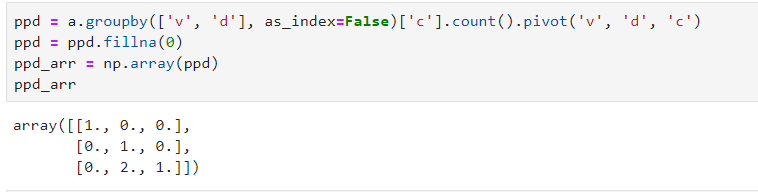总结一句话
np.array(a.groupby(['v', 'd'], as_index=False)['c'].count().pivot('v', 'd', 'c').fillna(0))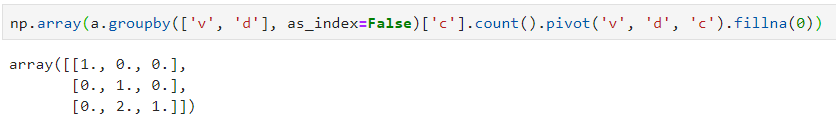第三种方法：pivot_table
pivoted_df=pd.pivot_table(a[['v','d','c']],
values='c', index=['v'],
columns=['d'], aggfunc='count')
pivoted_df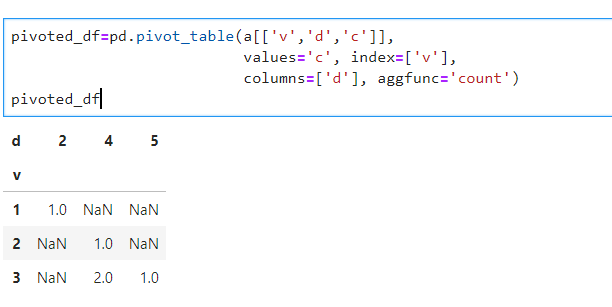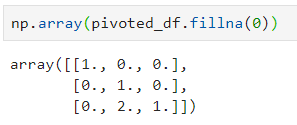展开全文python
• 统计总数 只要2中有n，这所有对应1的所有数据显示 也就是说只显示结果为一条记录 我之前写的sql语句，因为数据比较多，执行起来性能太差 SELECT COUNT(DISTINCT 1) AS 数量 FROM 表A AS a WHERE 1 NOT ...mysql select sql 性能 数据
• 已经建立好个表，但是题上要求用SQL语句的alter将设置非空，我用了alter table school modify colimn sno char not null但是出现error 1265(01000):data truncayed for column 'sno' at row 1 知道错在...sql
• 当字段类型文本时Varchar，超出1000数据时，出的最大值999，针对这个问题，解决方法是将文本转换成数值型在进行值，出的结果则正确 SQL语句： select max( TO_NUMBER(t.id))  from MDFIELD t ...
当字段类型为文本时Varchar，超出1000数据时，求出的最大值为999，针对这个问题，解决方法是将文本转换成数值型在进行求值，求出的结果则为正确

SQL语句：  select max( TO_NUMBER(t.id))   from MDFIELD t

应该注意的是：
使用TO_NUMBER()函数的时候，一定要确保所转换字段是可转换为数字的，比如字符串“20170720”是可以转换为数字20170720的，但是字符串“2017-07-20”不可以。如果字段中包含了字符串“2017-07-20”，并且还使用了TO_NUMBER()函数进行操作的话就会报“invalid number”的错。 
展开全文oracle sqlpython
• 对于这种，读进来日期不是日期格式，并且有时候天有很多条数据，天没有数据的情况，根据年份合并star_rating这栏的平均值。 读入数据并删除无关 pcfr = pd.read_excel('microwave.xlsx') df = pcfr[['...
对于这种，读进来日期不是日期格式，并且有时候一天有很多条数据，一天没有数据的情况，根据年份合并求star_rating这一栏的平均值。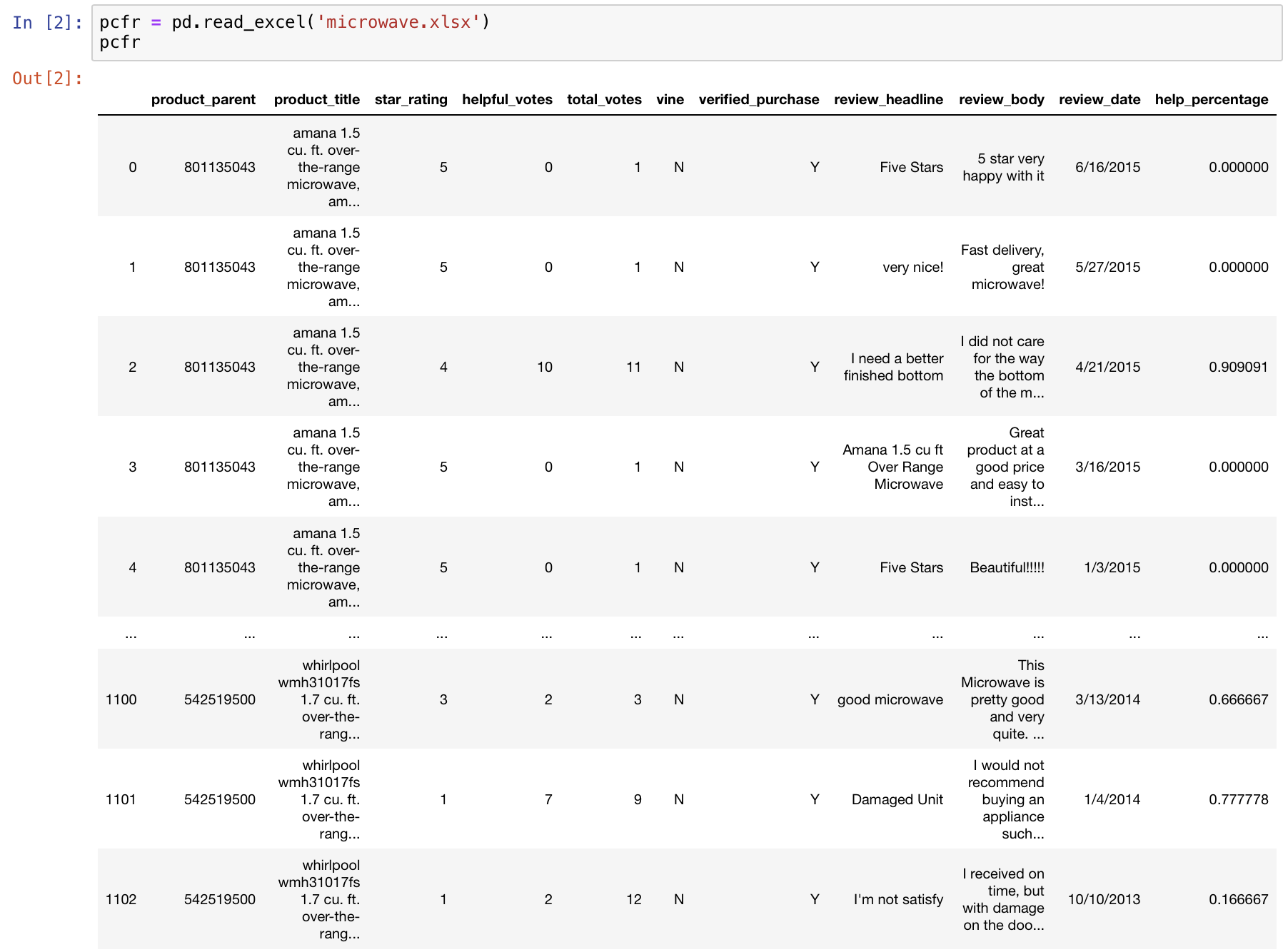读入数据并删除无关列
pcfr = pd.read_excel('microwave.xlsx')
df = pcfr[['review_date','star_rating']]

会警告，无视之。
df['review_date'] = pd.to_datetime(df['review_date'])

日期格式的转化
# df.set_index('review_date')
# df.reset_index()
t = df.set_index('review_date')

这一步结束后可以看到日期已经转换成功。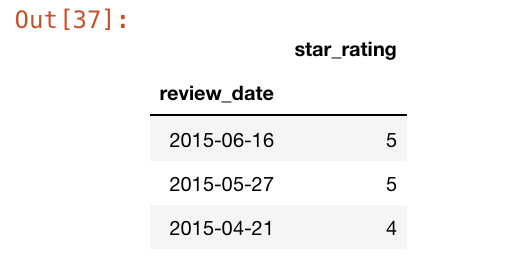利用groupby分组求年平均值
m = t.groupby(pd.Grouper(freq='Y')).mean()
print(m)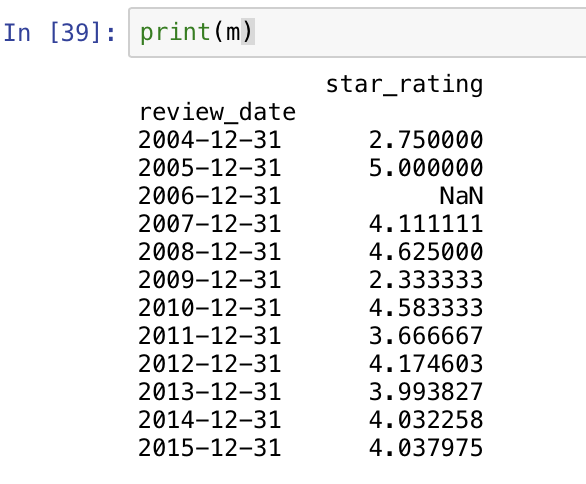展开全文python pandas
• 我在进行调试时，控件的datasource显示的是7列，但最终在界面显示时，只显示了6列，知道问题出在哪里，而且显示第一列解答c# vs2010
•  在matlab中求一个矩阵中元素的可以自己编写for循环来完成，这样比较方便，想那些数据的都可以做到，然而效率比较低，如果数据量大程序会跑好长时间。所以我们可以转而用matlab提供的sum函数。  设M为一个...

matlab中矩阵元素求和、求期望和均方差
wine(1:59,:)%提取前59行的所有列向量的子矩阵。
在matlab中求一个矩阵中元素的和可以自己编写for循环来完成，这样比较方便，想求那些数据的和都可以做到，然而效率比较低，如果数据量大程序会跑好长时间。所以我们可以转而用matlab提供的sum函数。
设M为一个矩阵，那么：
1、求和
sum（M）:以矩阵M的每一列为对象，对每一列的数据分别求和。
sum（M,2）:以矩阵的每一行为对象，对每一行的数据分别求和。
sum（M（：））：将矩阵中的所有元素相加求和。
2、求期望
matlab中矩阵元素求期望的函数mean与sum用法雷同。
mean（M）：以矩阵M的每一列为对象，对每一列的数据分别求期望。
mean（M,2）:以矩阵的每一行为对象，对每一行的数据分别求期望。
mean（M（：））：以矩阵所有数据为对象求期望。
3、求均方差
若要求整个矩阵所有元素的均方差，则要使用std2函数：std2（M）
注：Matlab中有求数组方差的 函数：var；要注意的是var函数所采用公式中，分母不是length（X） ，而是length（X）-1 。这是因为var函数实际上求的并不是方差，而是误差理论中“有限次测量数据的标准偏差的估计值”。var没有求矩阵的方差功能，可使用std先求均方差，再平方得到方差。std，均方差，std(X,0,1)求列向量方差，std(X,0,2)求行向量方差。
eg：
>>X=[1,2,3,4]
>>var(X)=1.6667
>> sum((X(1,:)-mean(X)).^2)/length(X)=1.2500
>> sum((X(1,:)-mean(X)).^2)/(length(X)-1)=1.6667

展开全文matlab Matlab
• 怎么第四的平均数呢？ Python版本 我们可以用几行Python代码解决，比如这样： 其耗时： R版本 用R来做计算也是很适合的，比如像这样： 其耗时： awk版本 awk用行代码就可以解决问题，像这样（注意耗时）：...awk
• =SUMPRODUCT(1/COUNTIF(A:A,A2:A6461)) 以上查询EXCEL中某一列不重复的值的计算公式 本例查的是A列中A2到A6461行之间所有值中重复的数量。Excel
• r矩阵某一列的标准偏差Being a statistical language, R offers standard function sd(‘ ‘) to find the standard deviation of the values. 作为一种统计语言，R提供标准函数sd（''）以查找值的标准偏差。 ...python 机器学习 数据分析 大数据 java
• 以o2o大赛的数据例 df_new = df[['User_id','Merchant_id']] df_new_1 = df_new.groupby(['User_id'])['Merchant_id'].nunique() 在同个'User_id'下，'Merchant_id'有多少个 df_new_1 = df_new.group...
• 由于最近在做项目的时候，要对数据库中行很多个数据进行求和，便在网上查了一下，首先要用到动态SQL语句，动态sql感觉就是类似于拼接sql字符串 动态语句基本语法 1 :普通SQL语句可以用exec执行 Select * ...sql server 动态SQL语句 syscolumns 合并
•  import numpy as np import pandas as pd df = pd.DataFrame(np.random.rand(10, 2), columns=list('ab')) df['a'] = 1 df['a'].iloc[5:] = 2 df[df['a']==2]['b'] = 10python
• 使用left（）函数实现。具体使用方法left（col_name，N），其中col_name列名，N左起前N个字符。 ...因为jine在设计数据表时数据类型varchar，sum函数可以直接使用，要先把varchar改...
• ## numpy求每列或每行中的最值、和

万次阅读 多人点赞 2020-03-06 17:19:34
一列中的最大值、最小值、每一列 numpy.max(A, axis=0) numpy.min(A, axis=0) numpy.sum(A, axis=0) a = numpy.arange(12) A = a.reshape((3, 4)) print(A) print("--"*20) print(numpy.max(A, axis=0)) ...
• //给定个5*5的矩阵，每行只有个最大值，每只有个最小值，寻找这个矩阵的鞍点。 //鞍点指的是矩阵中的个元素，它是所在行的最大值，并且是所在的最小值。 //例如：在下面的例子中（第4行第1的元素就是...
• python对矩阵中的某一列取整，有没有什么函数可以直接，而不是写for循环啊python 取整
• C# DataTable 某一列求和 1. 列为数字类型 double total= Convert.ToDouble(datatable.Compute("SUM(需要求和的参数)", "")); 2.列为string 类型 先转为数字类型 再求和 （遇到是采用了这个方法） 会报错，加using ...c#
• 一列数的最后一个数往往用于计算合计数或代表整列某个性质的值，所以往往需要取这个数，然而有时这个数是在列中固定的行上。所以需要取一列数的最后一个非空的值的函数 Excel 所有版本最大行，列数excel office
• 一列的元素 获取某一个元素 获取某一行元素 获取某一列元素 获取某一范围元素 import numpy as np a=np.random.random((3,4))#随机生成3行4列小数数组 #b=np.random.randint(0,10,size=[3,4])#随机生成3行4列...python numpy 列表
• JasperReport，如何利用sum计算某一列的值，重点是只计算当前页，其他页的计算！ 大神指教，谢谢！
• 在excel中如何将一列很长的数据平均分成几列 方法一： 参考网址：https://zhidao.baidu.com/question/522133495.html?qbl=relate_question_0 假设你说的一列是A列 B1单元格输入公式 =OFFSET($A$1,ROW(A1)-1+...Excel OFFSET
• 个n*m的矩阵，其中同行并且不同的两个数的最大乘积。 n,m<1000 分析： 首先矩阵不大，1000*1000，一共1000000个元素，我先想到了排序，从大到小把所有元素排序，然后选择最大的两个。 这两个如果...
• 总共有接近七千行数据，主要的工作就是将其中的股价数据提取出来，放入个数组之中，然后利用numpy模块即可出需要的数据。 这里利用了csv模块来对文件进行处理，最终实现的代码如下： import csv import numpy as...python csv 数据分析 numpy 大数据
• 原来是字符串格式，要改datetime格式 from datetime import datetime import pandas as pd 方法：df[‘date’].astype(‘datetime64’) tran['date'] = tran['date'].astype('datetime64[ns]') 方法二：pd.to_...
• %b是个2行3的矩阵  4 5 6] b(1,:)= [] % 删除第行 b(:,2)= []  % 删除第二 b(3)= [] %删的是元素2，按来数，删完后，b就变为个行向量 2 how 追加向量 b=[1 ...matlab
• 多说，先看怎么修改某一列的最大值。 那首先就要先找到该列的最大值： import numpy as np import pylab as plt plt.style.use("ggplot") import pandas as pd df = pd.DataFrame({"A":[1,2...loc
• 需求 从excel读取数据用DataFrame存储， 1. 逐行处理，当指定多的值满足一定条件时，取这几列的最大值作为...对于赋值来自原有字段的，先赋值数字，便于后续计算，再按条件修改 #增加字段rs_flag,rs_value .python
• 需求现在有个文件格式如图ID 1 2 3 4 5 6 7 8 9 10 11 12 13 14 15 16 17001 89.84 8.87 1.29 -0.0 0.0 68.99 0.0 0.0 4.67 1.48 0.0 0.0 14.69 0.0 0.0 0.0 0.01002 0.0 0.0 0.0 0.0 0.0 0.0 0.0 0.0 99.99 0.0 ......